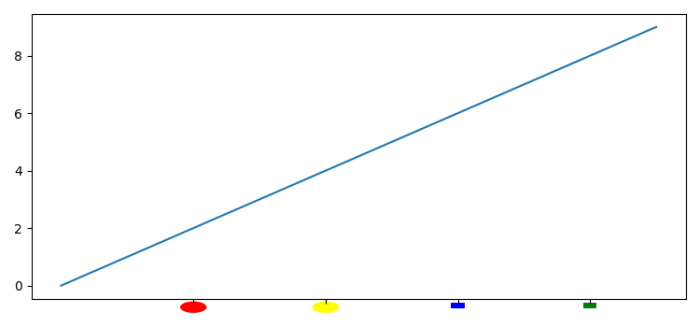# How can I make the xtick labels of a plot be simple drawings using Matplotlib?

To make xtick labels of a plot be simple drawings using Matplotlib, we can take the following steps −

• Set the figure size and adjust the padding between and around the subplots.
• Initialize the y position of simple drawings.
• Create a new figure or activate an existing figure using figure() method.
• Add an '~.axes.Axes' to the figure as part of a subplot arrangement using add_subplot()method.
• Plot a line using plot() method.
• Set the X-axis ticks using set_ticks() method.
• Set empty tick labels.
• Add circles and rectangles patches using add_patch() method. Instantiate Circle() and Rectangle() class.
• To display the figure, use show() method.

## Example

import matplotlib.pyplot as plt
import matplotlib.patches as patches

plt.rcParams["figure.figsize"] = [7.50, 3.50]
plt.rcParams["figure.autolayout"] = True

y = -.75
fig = plt.figure()

ax.plot(range(10))

ax.get_xaxis().set_ticks([2, 4, 6, 8])
ax.get_xaxis().set_ticklabels([])

clip_on=False, facecolor='red'))
plt.show()